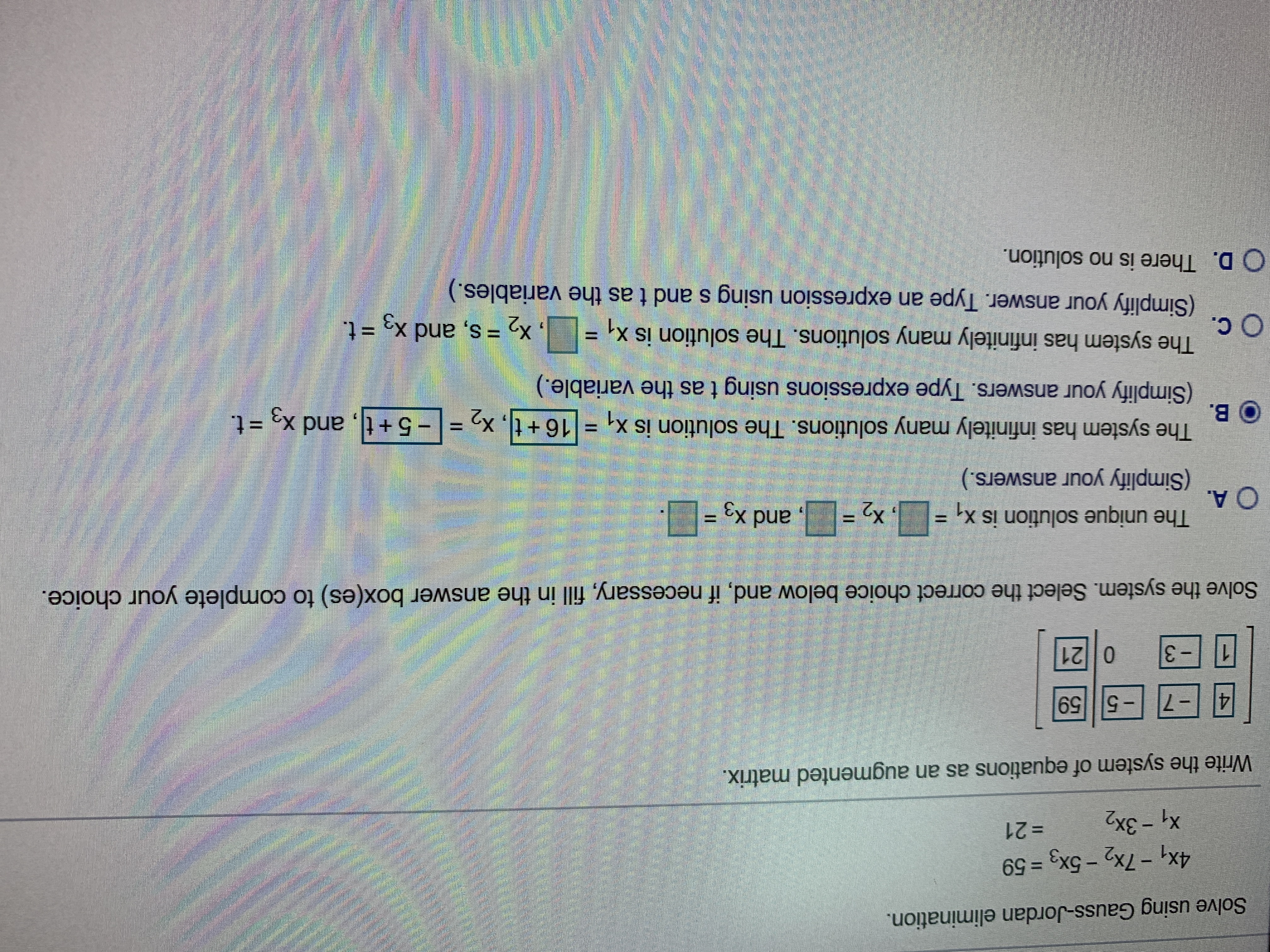# Solve using Gauss-Jordan elimination. 4x1-7x2-5x3 59 X1-3x2 =21 Write the system of equations as an augmented matrix. - 7559 4 21 0 3 Solve the system. Select the correct choice below and, if necessary, fill in the answer box(es) to complete your choice. The unique solution is x, ,X O A. (Simplify your answers.) ,and x3 = The system has infinitely many solutions. The solution is x, =16+t В. -5+t, and x =t. X2 (Simplify your answers. Type expressions using t as the variable.) The system has infinitely many solutions. The solution is x. Ос. X2 = S, and x3 = t. (Simplify your answer. Type an expression using s and t as the variables. D. There is no solution.

Question

Can you be more clear of what the answer choice is and to put in the blanks please.help_outlineImage TranscriptioncloseSolve using Gauss-Jordan elimination. 4x1-7x2-5x3 59 X1-3x2 =21 Write the system of equations as an augmented matrix. - 7559 4 21 0 3 Solve the system. Select the correct choice below and, if necessary, fill in the answer box(es) to complete your choice. The unique solution is x, ,X O A. (Simplify your answers.) ,and x3 = The system has infinitely many solutions. The solution is x, =16+t В. -5+t, and x =t. X2 (Simplify your answers. Type expressions using t as the variable.) The system has infinitely many solutions. The solution is x. Ос. X2 = S, and x3 = t. (Simplify your answer. Type an expression using s and t as the variables. D. There is no solution. fullscreen

### Want to see the step-by-step answer?